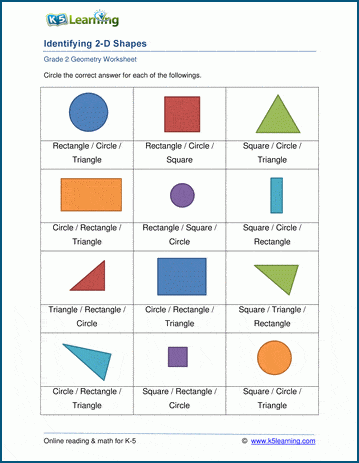# Geometry Worksheets

## Geometry and shapes worksheets

Our geometry worksheets start with introducing the basic shapes through drawing and coloring exercises and progress through the classification and properties of 2D shapes including quadrilaterals, triangles, circles, and polygons.  Area and perimeters, classification of angles, and plotting on coordinate grids are also covered.  Latter worksheets introduce 3D shapes.

Kindergarten shapes worksheets

Topics include:

## Kindergarten shapes worksheets

• Tracing and drawing the basic shapes (2D)
• Matching similar shapes
• Matching shapes to their names
• Identifying basic 2D shapes
• Drawing shapes in a pattern
• Is the shape 2D or 3D?

• Matching similar shapes
• Matching shapes to their names
• Identifying and labeling 2-D shapes
• Drawing and identifying squares, rectangles, triangles, circles, ovals, diamonds
• Rotating 2D shapes
• Scaling 2D shapes

• Identifying 2D shapes including pentagons, hexagons
• Counting edges and vertices
• Comparing edges and vertices of different shapes
• Composing and decomposing 2D shapes
• Identifying parts of a whole using shapes
• Congruent shapes
• Lines of symmetry
• Drawing symmetric shapes
• Area and perimeter of 2D shapes
• Area of a rectangle on a rectangular grid
• Perimeters of common shapes
• Identifying 3D shapes
• Matching 3D shapes to real objects
• Counting edges, faces and vertices
• Comparing edges, faces and vertices of different shapes

• Matching quadrilaterals to their names
• Identifying parallelograms
• Classifying triangles by their angles (acute / obtuse / right)
• Classifying triangles by their sides (scalene, isosceles, equilateral)
• Properties of circles
• Properties of polygons
• Regular polygons
• Lines, segments and rays
• Comparing angles and right angles
• Parallel and perpendicular lines
• Classifying angles
• Measuring angles
• Drawing angles with a protractor

• Classifying angles
• Classifying triangles
• Perimeter and area of rectangles
• Perimeter and area of irregular rectangular shapes
• Circumference of a circle

• Classify and measure angles
• Classify triangles
• Area and perimeter of rectangles (customary units, metric)
• Area and perimeter of irregular rectangular shapes (customary units, metric)
• Area of right triangles
• Area of triangles
• Area of triangles, parallelograms and trapezoids
• Circumference of a circle
• Area of a circle
• Volume and surface area of rectangular prisms
• Plotting and reading points on a grid

• Classify and measure angles and triangles
• Area and perimeter of irregular rectangular shapes
• Area and perimeter of triangles and quadrilaterals
• Area and perimeter of parallelograms and trapezoids
• Circumference and area of circles
• Volume and surface area of rectangular prisms (fractional lengths, decimals)
• Volume and surface area of 3D shapes

## Related topics

Fractions worksheets

Measurement worksheetsSample Geometry Worksheet

What is K5?

K5 Learning offers free worksheets, flashcards and inexpensive workbooks for kids in kindergarten to grade 5. Become a member to access additional content and skip ads.